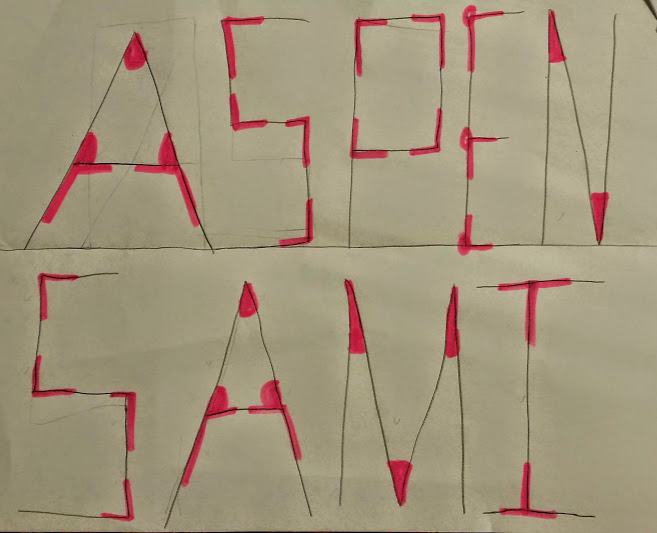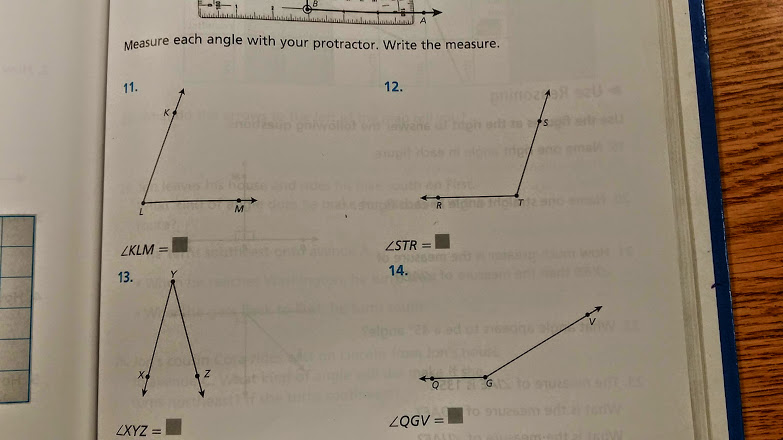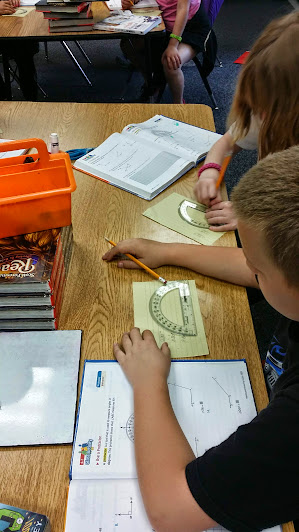# Practice with Protractors

9 teachers like this lesson
Print Lesson

## Objective

SWBAT use a protractor to measure angles to the nearest degree.

#### Big Idea

In this direct instructional approach lesson, students practice using a protractor to measure various sized angles.

## Warm Up

6 minutes

For this warm up, I wanted to review the definitions of acute, obtuse, and right angles.  I direct students to write their names in block style lettering in all capital letters. I tell students to write their name on one half of a plain piece of 8 x 11 piece of paper and and friends name on the other half.   Once students write the names, they must identify the angles in the names.  Students identify the angles by coloring or shading them in and keeping a tally of how many of each type of angle they have.

I also assign point values to each type of angle and ask students to find the total point value for their names.  Acute angles are 500 points each, obtuse angles are 1000 points each, and right angles are 700 points each.

In this photo, you can see how a student identified the various angles in her name by shading them in.## Concept Development

45 minutes

To begin this lesson, I show students this interactive smart board page - protractor

I show students the two different kinds of protractors - half circle and circle protractor.  I lead a discussion about how they are similar to the pattern blocks and wedges we used in previous lessons.

Next, I demonstrate the steps to measuring angles with a protractor using the half circle shaped protractor.

1. Find the vertex on the straight edge of the protractor.

2. Place the hole, or vertex in the middle of the straight edge on the protractor, over the vertex of the angle you are measuring.

3. Line up the zero on the straight edge of the protractor with one of the sides of the angle.

4. Find the place where the second side of the angle intersects the curved edge of the protractor.

5. Read the number that is written on the protractor at the point where the line intersects. Be sure to start from zero and skip count by 10’s, then 5’s. This is the measure of the angle in degrees (to the nearest 5)

Note: The above directions are what I tell my students, but I also lead a discussion and show many examples of how to use the protractor with out lining up the zero line on the ray of the angle. I want my students to realize that they can use the inside or outside numbers to find the degree of an angle.

For the next part of the lesson, students practice using their protractor and measure angles from a page in a math book or worksheet. Below are some angles my students measured.Once students have measured angles, I direct them to read a page from the math book that explains how to also use the protractor to draw angles.  I then ask students to create a few angles of their own.

In this photo, you can see students measuring angles in their book and also drawing angles and exploring protractor use.Listen in as this student struggles to measure an angle with a protractor and my approach to guide this student in the right direction.

Listen in as this students wrestles with the idea that he thought he drew a 95 degree angle, but when I ask him about it, he realizes that his angle is not 95 degrees because it is not obtuse.  You can hear the hesitation in his voice and how he is just beginning to use logic to make sense of whether or not he drew the correct angle.